Home MonkeyNotes Printable Notes Digital Library Study Guides Study Smart Parents Tips College Planning Test Prep Fun Zone Help / FAQ How to Cite New Title Request

5.12 Concavity and Points of Inflection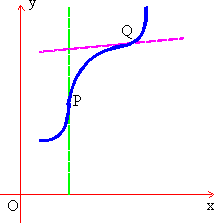The second derivative of a function may be also be used to determine the general shape of y = f (x) on a selected interval.

A point of inflection on a curve is a point where the curve changes its direction of curvature. See the adjoining fig. You will notice that at P the curve changes from being concave upward to being concave downwards. At Q, it changes from concave downwards to being concave upwards. Since f " (x) i.e.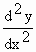is the rate of change of the gradient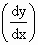, a graph is concave upwards where f " (x) > 0 and concave downwards where f " (x) < 0.

Now at a point of inflection, the gradient stops increasing and begins decreasing, or it stops decreasing and begins increasing. Thus a point of inflection is one at which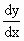is either a maximum or a minimum. Thus the condition for a point of inflection is that= 0 at the point and it changes sign in passing through it i.e.= 0 and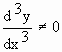Note that , the tangent to a curve at a point of inflection crosses the curve, or more accurately, it cuts the curve at three coincident points.Your browser does not support the IFRAME tag.

Problems On Maxima And Minima With Concavity Of A Curve

Example 23

Determine the concavity of y =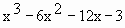and identify any point of inflection.

Solution : Since f (x) is a polynomial in x, x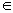R

Now f ' (x) =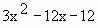and f " (x) = 6x - 12

f "(x) = 0

Þ 6x - 12 = 0

\ 6x = 12

\ x = 2

We find that f " (x) < 0 on ( - ¥, 2) ® Left of x = 2

and f " (x) > 0 on (2, ¥ ) ® Right of x = 2

Hence f(x) is concave downwards on (- ¥, 2) and concave upwards on (2, ¥) and f (x) has a point of inflection at (2, - 38)

Index

5.1 Tangent And Normal Lines
5.2 Angle Between Two Curves
5.3 Interpretation Of The Sign Of The Derivative
5.4 Locality Increasing Or Decreasing Functions 5.5 Critical Points
5.6 Turning Points
5.7 Extreme Value Theorem
5.8 The Mean-value Theorem
5.9 First Derivative Test For Local Extrema
5.10 Second Derivative Test For Local Extrema
5.11 Stationary Points
5.12 Concavity And Points Of Inflection
5.13 Rate Measure (distance, Velocity And Acceleration)
5.14 Related Rates
5.15 Differentials : Errors And Approximation